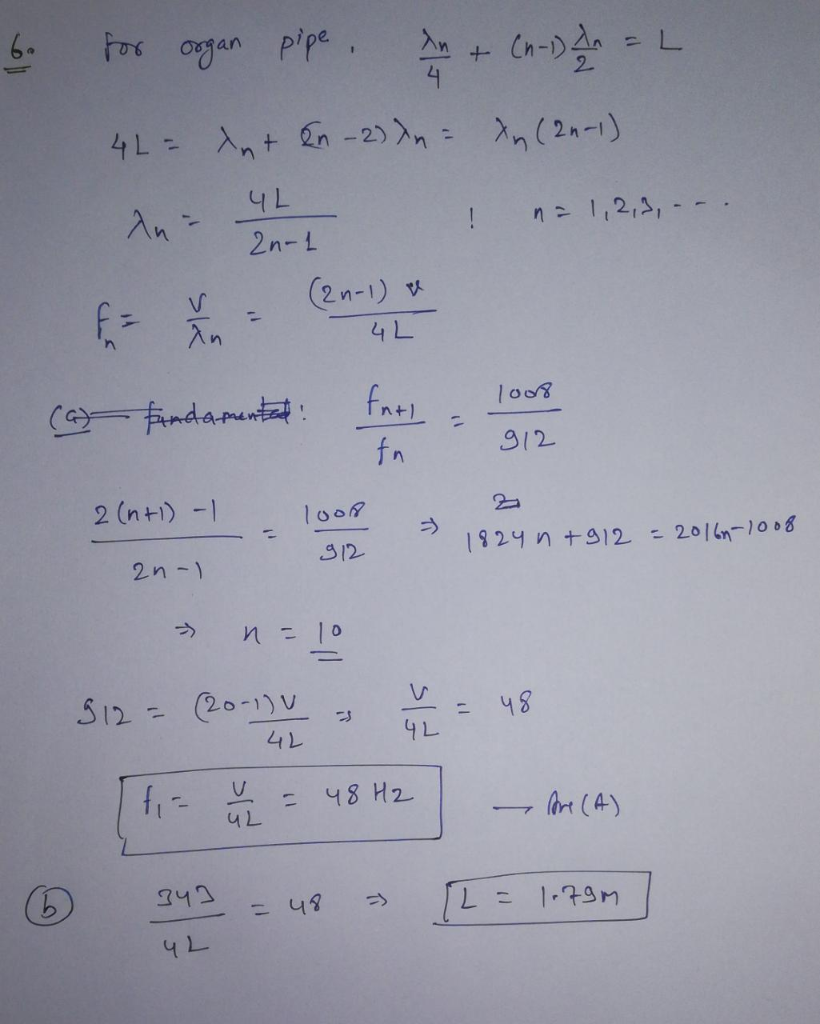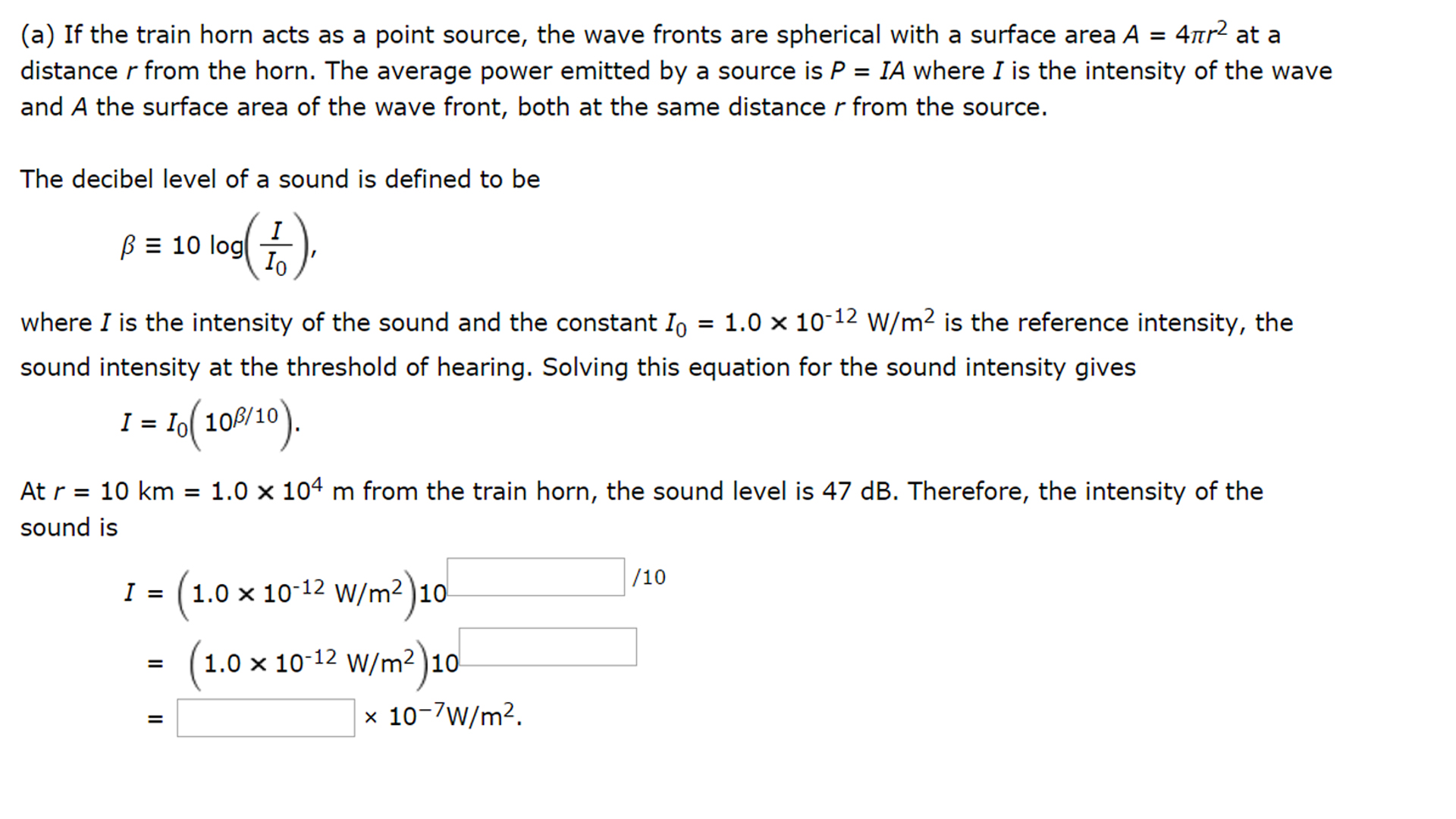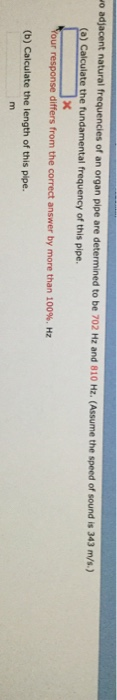Question

# 6)Two adjacent natural frequencies of an organ pipe are determined to be 912 Hz and 1008...

6)Two adjacent natural frequencies of an organ pipe are determined to be 912 Hz and 1008 Hz. (Assume the speed of sound is 343 m/s.)

(a) Calculate the fundamental frequency of this pipe.

(b) Calculate the length of this pipe.

7)A train sounds its horn as it approaches an intersection. The horn can just be heard at a level of 60 dB by an observer 10 km away.

(a) What is the average power generated by the horn?

(b) What intensity level of the horn's sound is observed by someone waiting at an intersection 54 m from the train? Treat the horn as a point source and neglect any absorption of sound by the air.#### Earn Coins

Coins can be redeemed for fabulous gifts.

Similar Homework Help Questions
• ### A train sounds its horn as it approaches an intersection. The horn can just be heard...A train sounds its horn as it approaches an intersection. The horn can just be heard at a level of 47 dB by an observer 10 km away. (a) What is the average power generated by the horn? (b) What intensity level of the horn's sound is observed by someone waiting at an intersection 43 m from the train? Treat the horn as a point source and neglect any absorption of sound by the air.

• ### a train sounds its horn as it approaches an intersection. The horn can just be heard...

a train sounds its horn as it approaches an intersection. The horn can just be heard at a level of 40dB by an observer 10 km away. a) what is the average power generated by the horn? b) what intensity level of the horn's sound is observed by someone waiting at an intersection 58m from the train? Treat the horn as a point source and neglect any absorption of sound by the air.

• ### frequencies of an organ pipe are determined to be 702 Hz and 810 HE. (Assume the...frequencies of an organ pipe are determined to be 702 Hz and 810 HE. (Assume the speed of sound is 343 m/s.) a) Calculate the fundamental frequency of this pipe xt our response differs from the correct answer by more than 100%. Hz (b) Calculate the length of this pipe.

• ### 7)A train sounds its horn as it approaches an intersection. The horn can just be heard...

7)A train sounds its horn as it approaches an intersection. The horn can just be heard at a level of 60 dB by an observer 10 km away. (a) What is the average power generated by the horn? Answer must be in W (b) What intensity level of the horn's sound is observed by someone waiting at an intersection 54 m from the train? Treat the horn as a point source and neglect any absorption of sound by the air....

• ### Two trains approach each other on separate but adjacent tracks. Train 1 is traveling at a...

Two trains approach each other on separate but adjacent tracks. Train 1 is traveling at a speed of 35.9 m/s and train 2 at a speed of 27.6 m/s. If the engineer of train 1 sounds his horn which has a frequency of 520 Hz, determine the frequency of the sound heard by the engineer of train 2. (Use 343 m/s as the speed of sound. Enter your answer to the nearest Hz.)

• ### An organ pipe is observed to produce 3 consecutive harmonics with frequencies of 189, 243 and...

An organ pipe is observed to produce 3 consecutive harmonics with frequencies of 189, 243 and 297 Hz. By how much does the tube need to be shortened so that the fundamental frequency is 60 Hz? The speed of sound is 343 m/s.

• ### 1. A bystander hears a siren vary in frequency from 586 Hz to 392 Hz as...

1. A bystander hears a siren vary in frequency from 586 Hz to 392 Hz as a fire truck approaches, passes by, and moves away on a straight street. What is the speed of the truck? (Take the speed of sound in air to be 343 m/s.) Answer in 3 significant figures m/s 2. A trailer truck traveling east at 34.0 m/s sounds a 1200 Hz horn. What is the wavelength of the sound heard by a stationary listener located...

• ### An open pipe on an organ creates a fundamental frequency at 10500 Hz. How long is...

An open pipe on an organ creates a fundamental frequency at 10500 Hz. How long is the pipe (speed of sound=343 m/s, unit=m)?

• ### A train is moving parallel and adjacent to a highway with a constant speed of 32 m/s.

A train is moving parallel and adjacent to a highway with a constant speed of 32 m/s. A car is traveling in the same direction as the train at 64 m/s. The car's horn sounds at 520 Hz and the train's whistle sounds at 320 Hz. When the car is behind the train what frequency does an occupant of the car observe for the train whistle? The speed of sound is 343 m/s. Answer in units of Hz. When the car is in...

• ### Doppler Effect You are waiting at a train station when an approaching express train sounds its...

Doppler Effect You are waiting at a train station when an approaching express train sounds its 400 Hz horn, warning everyone to stand back from the platform as it passes. If you perceive the frequency of the horn to be 425 Hz as the train approaches, how fast must the train be moving towards you? You may assume the speed of sound is 343 m/s.